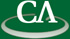A Sparse Strategy for Polynomial Division with Application to F4.

Roman Pearce, CECM

Thursday June 8th, 2006 at 11:30am in K9509.

```Abstract:

We will introduce a sparse strategy for multivariate polynomial division
based on linear algebra and the F4 algorithm for computing Groebner bases.
Like F4, our method constructs a large sparse linear system to efficiently
reduce several polynomials at once.  Unlike F4, our linear system is block
triangular, so it can be solved using back substitution and a topological
sort.  The resulting algorithm constructs the terms of the remainder(s)
directly as linear combinations of irreducible terms.  Finally, we will
demonstrate an improved F4 algorithm based on this technique.

```